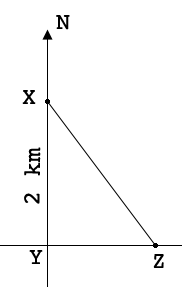SEARCH HOMEMath Central Quandaries & QueriesQuestion from Jean: X is due north of Y and 2km distant. Z is due east of Y and has a bearing of S35°12'E from X. How far, to the nearest metre, is Z from X? Will you help me solve this problem?Hi Jean,

I would first draw a diagramA bearing of $S 35^o 12'$ means that $Z$ is 35 degrees 12 seconds east of south so the measure of angle $ZXY$ is $35^o 12'.$ 12 minutes is $\large \frac{12}{60} \normalsize = 0.2$ degrees so if you prefer angle measures in decimal fractions the measure of the angle $ZXY$ is $35.2^{o}.$

You want to know the distance from $Z$ to $X.$ What trig function relates the length of $ZX,$ the length of $XY,$ and the measure of the angle $ZXY?$

HarleyMath Central is supported by the University of Regina and The Pacific Institute for the Mathematical Sciences.